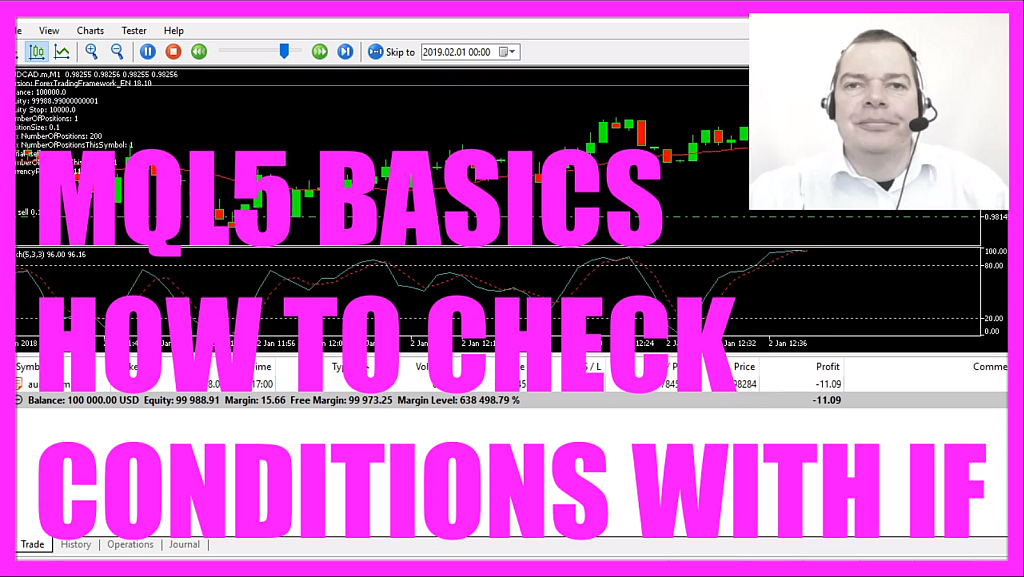In this video we are going to find out if some condition is true, whenever you use an automated program like this Expert Advisor here you obviously need to check if something is true or false, for example if the Stochastic is above or below the dotted lines here or if the price is above or below the Moving Average and now we want to find out how to do that.
Please click on a little icon here or press F4 and now you should see the Metaeditor and here you want to click on: “File/ New/ Expert Advisor (template)” from template, “Continue”, I will call this file: “SimpleIfCondition”, now click on “Continue”, “Continue” and “Finish”.
Now you can delete everything that is above the “OnTick” function and let’s also remove the two comment lines here, we are going to start by using two integer variables “a” is 5 and “b” should be 3 and if “a” is greater than 5 I would like to see the text: “a is greater than 5” and if that is not the case I can use the “else” statement, so whenever none of the conditions are true I would like to see the text: “Conditions not true”, so that’s about it.
Please click on the “Compile” button or press F7, we don’t have any errors here… Oh, I’ve got a warning because I forgot the “Comment” statement and now everything is okay, so let’s click on a little button here or press F4 to go back to Metatrader.
And in Metatrader we click on: “View/ Strategy Tester”, you could also press CTRL and R and here we want to pick the file: “SimpleIfCondition.ex5”, please enable the visualization mode here and start a test.
And now we get the output: “Conditions not true” because “a” is not greater than 5, so let’s add another “if” statement here where we check if “a” is equal to 5.
Now let’s recompile the code, stop the test, start a new one and this time the output is: “a equals 5”.
Now what will happen if we add another “if” statement here to check if “b” equals 3?
Let’s recompile the code, start another test and now we get only the output that “b equals 3”, the statement here is ignored and what we could do now is we could use two “if” statements. In the first one we will check if “a is 5”, the second one will check if “b equals 3” and the output will be: “a equals 5 and b equals 3”.
Let’s recompile the code and in our next test we will get the output “a equals 5 and b equals 3”.
So far so good but if you add anything here, for example we want that “c equals a plus b” and we want to output the value for c, so when we click on the “Compile” button now we get two errors here and the reason is that whenever we use more than one line behind the ”if” statement we need to use two curly braces, so let’s recompile the code and now it works and now in our last test we get the output: “c equals 8”.
This was a very basic example, there are a lot of other additional ways to check conditions but in this little video you have learned how to check if a condition is true by using the “if” statement and you have coded yourself with a few lines of MQL5 code.